# Linear algebra questions and answersRecent questions in Linear algebra
Vectors and spaces
ANSWERED### if x, y belong to R^p, than is it true that the relation norm (x+y) = norm (x) + norm (y) holds if and only if x = cy or y = cx with c>0

Vectors and spaces
ANSWERED### Vectors u and v are orthogonal. If u=<3, 1+b> and v=<5, 1-b> find all possible values for b.

Vectors and spaces
ANSWERED### The average height of a 2 year old boy is 38 inches. an 8 year old averages 56 inches. Use this information to write a linear equation that models the height (in inches), y, in terms of the age (in years), x. Use the linear equation to predict the average height of a 5 year-old boy.

Vectors and spaces
ANSWERED### $Find the minimum and maximum values of $$\displaystyle{y}=\sqrt{{6}}\theta-\sqrt{{3}}{\sec{\theta}}$$ on the interval PSK[0,\frac{\text{pi}}{\text{3}}]$

Forms of linear equations
ANSWERED### The reduced row echelon form of a system of linear equations is given.Write the system of equations corresponding to the given matrix. Use x, y. or x, y, z. or $$x_1, x_2, x_3, x_4$$ as variables. Determine whether the system is consistent or inconsistent. If it is consistent, give the solution. $$\begin{bmatrix}1 & 0 & 0 & 0 & 1\\ 0 &1 & 0 &0 & 2 \\ 0 & 0 & 1 & 2 & 3 \end{bmatrix}$$

Forms of linear equations
ANSWERED### Consider the system (*) whose coefficient matrix A is the matrix D listed in Exercise 46 and whose fundamental matrix was computed just before the preceding exercise.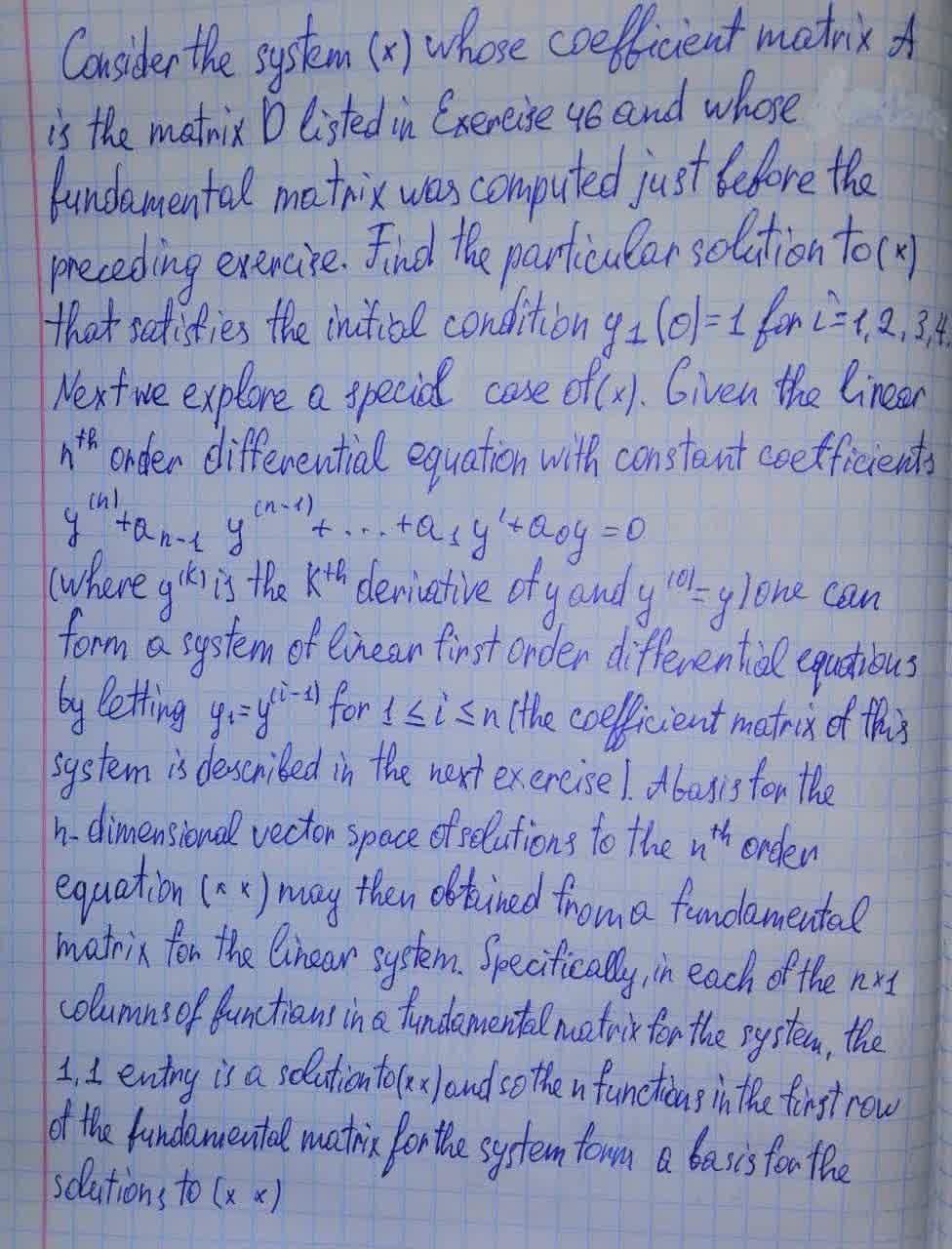Forms of linear equations
ANSWERED### Write the vector form of the general solution of the given system of linear equations. $$\displaystyle-{x}_{{1}}+{2}{x}_{{3}}-{5}{x}_{{4}}+{x}_{{5}}-{x}_{{6}}={0}$$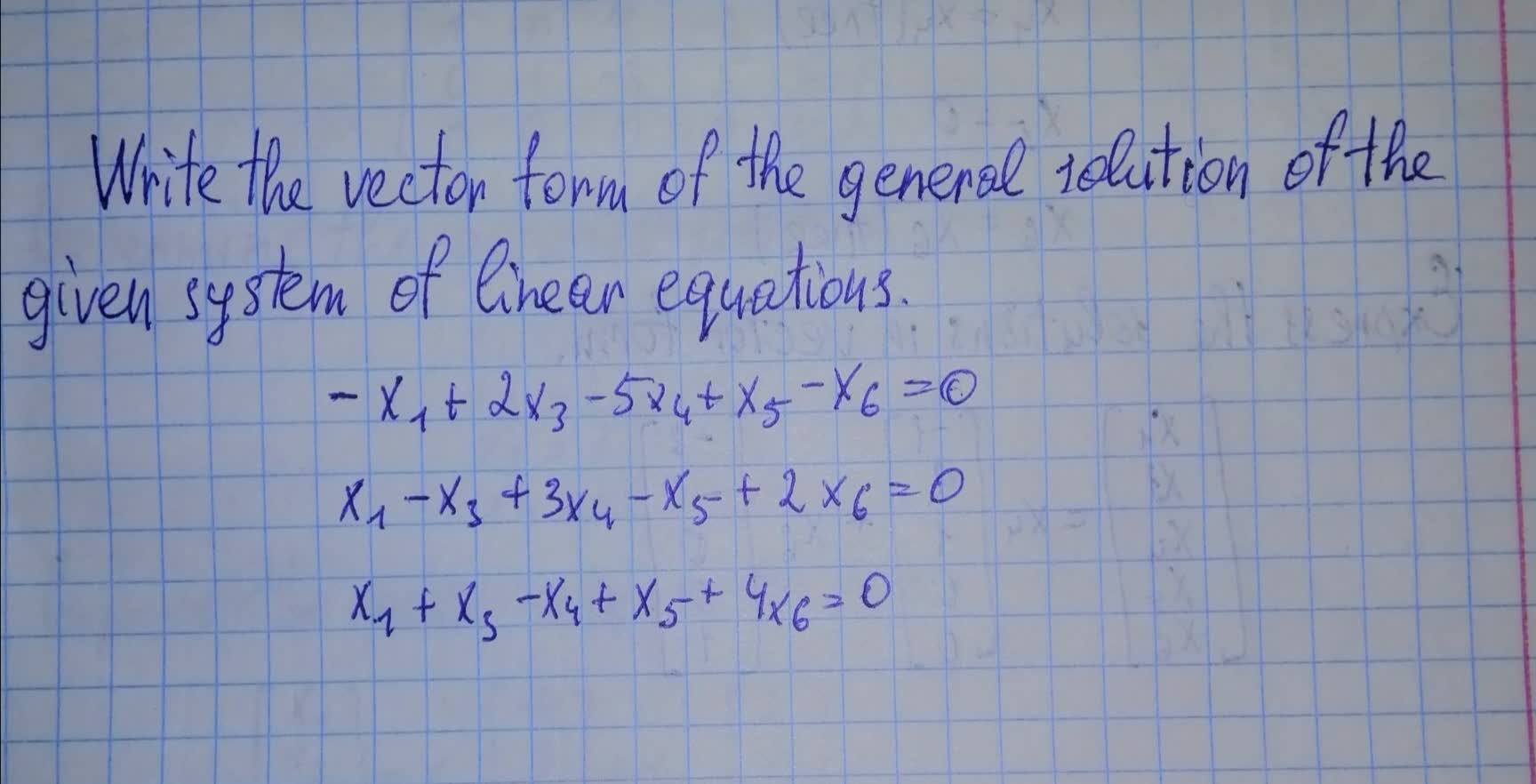Forms of linear equations
ANSWERED### The row echelon form of a system of linear equations is given. a) Write the system of equations corresponding to the given matrix. Use x, y. or x, y, z. or $$x_1,x_2,x_3,x_4$$ as variables. Determine whether the system is consistent or inconsistent. If it is consistent, give the solution. $$\begin{bmatrix}1 & 2 & -1 & 0 \\ 0 & 1 & -1 & 1 \\ 0 & 0 & 0 & 2 \end{bmatrix}$$

Forms of linear equations
ANSWERED### Write the vector form of the general solution of the given system of linear equations. $$\displaystyle{x}_{{1}}+{2}{x}_{{2}}-{x}_{{3}}+{2}{x}_{{5}}-{x}_{{6}}={0}$$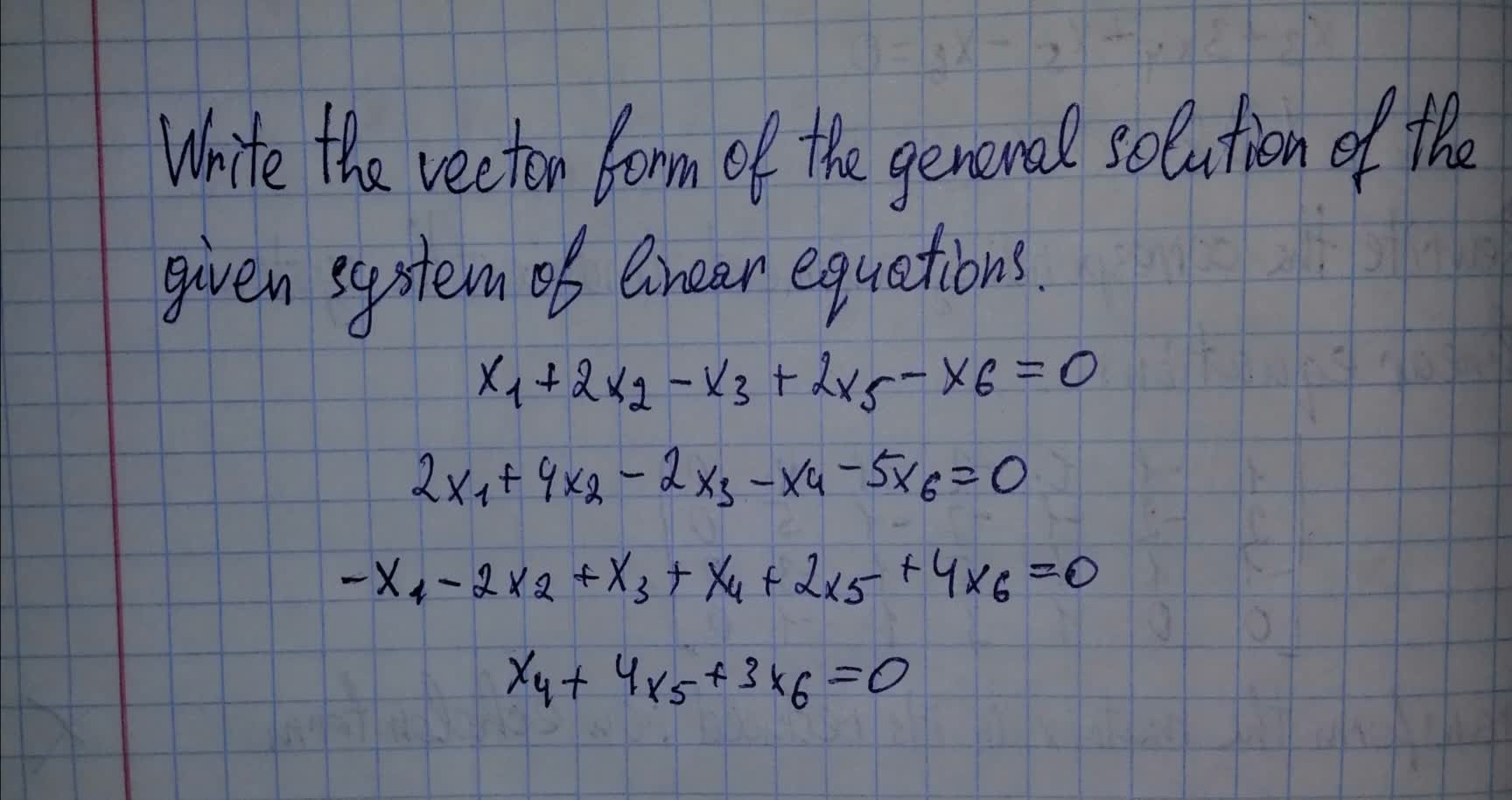Forms of linear equations
ANSWERED### The given matrix is the augmented matrix for a system of linear equations. Give the vector form for the general solution. $$\begin{bmatrix} {1}&{0}&-{1}&-{2}&-{3}&{1}\\{0}&{1}&{2}&{3}&{4}&{0}\end{bmatrix}$$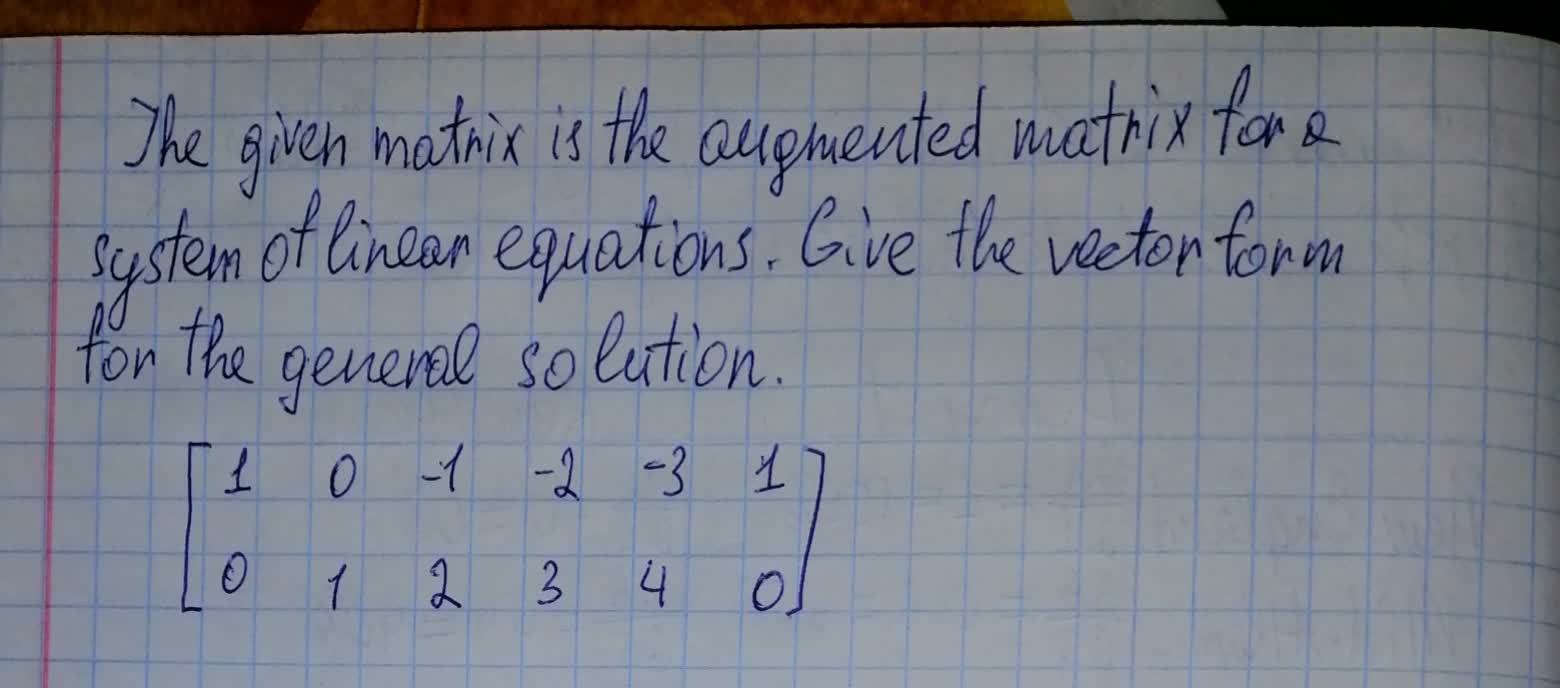Alternate coordinate systems
ANSWERED### Solve the following systems of inequalities graphically on the set of axes below.The state the following coordinates of a point in the solution say $$\displaystyle{y}\leq{x}+{6}$$ $$\displaystyle{y}\succ{\frac{{{3}}}{{{2}}}}{x}-{4}$$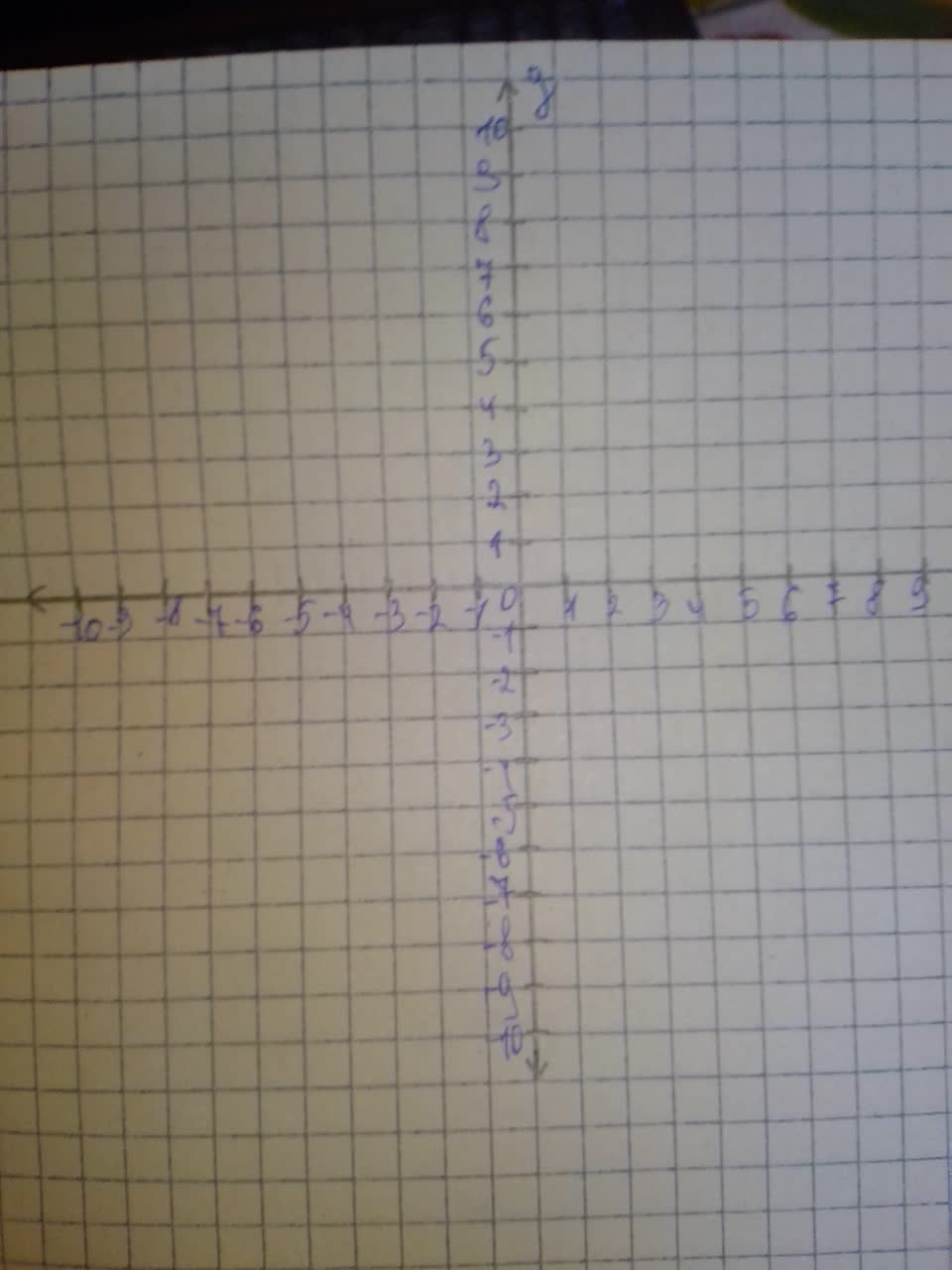Forms of linear equations
ANSWERED### The given matrix is the augmented matrix for a system of linear equations. Give the vector form for the general solution. $$\begin{bmatrix}{1}&{0}&-{1}&-{2}&-{3}&{0}\\{0}&{1}&{2}&{3}&{4}&{0}\end{bmatrix}$$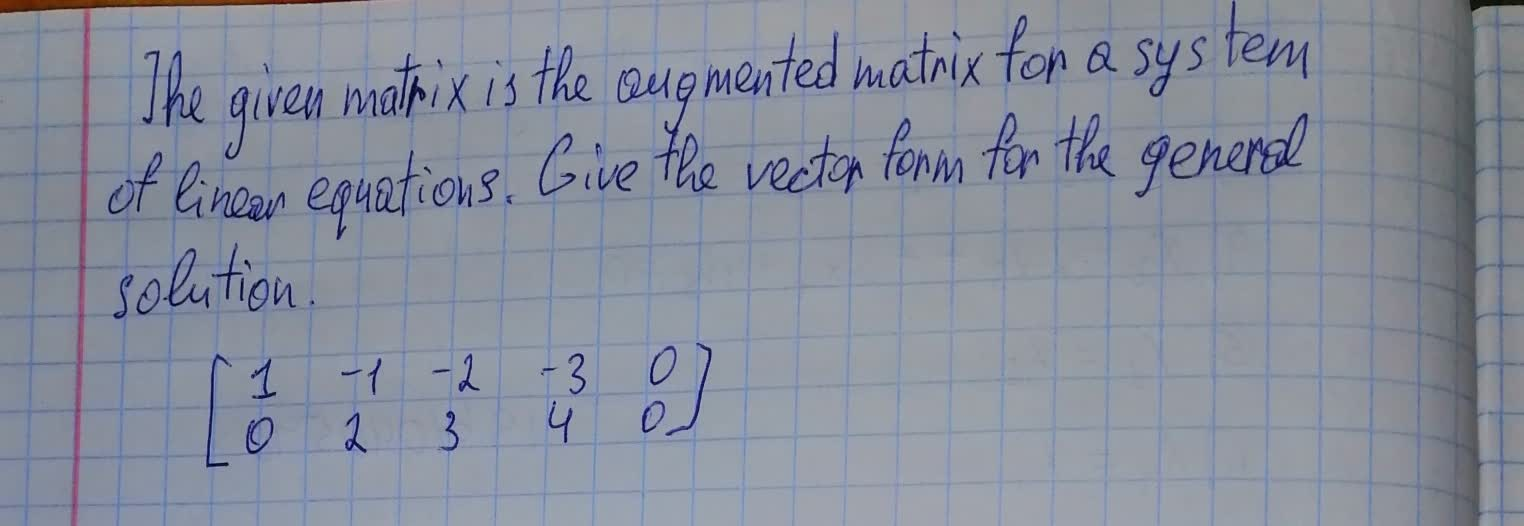Alternate coordinate systems
ANSWERED### Consider the following system. $$\begin{cases}{y}={30}-{x}\\{2}{y}=-{x}^{{{2}}}+{16}{x}-{12}\end{cases}$$

Forms of linear equations
ANSWERED### The reduced row echelon form of the augmented matrix of a system of linear equations is given. Determine whether this system of linear equations is consistent and, if so, find its general solution. $$\begin{bmatrix} {0}&{0}&{1}&-{3}&{0}&{2}&{0}\\ {0}&{0}&{0}&{0}&{1}&-{1}&{0}\\ {0}&{0}&{0}&{0}&{0}&{0}&{0}\\ \end{bmatrix}$$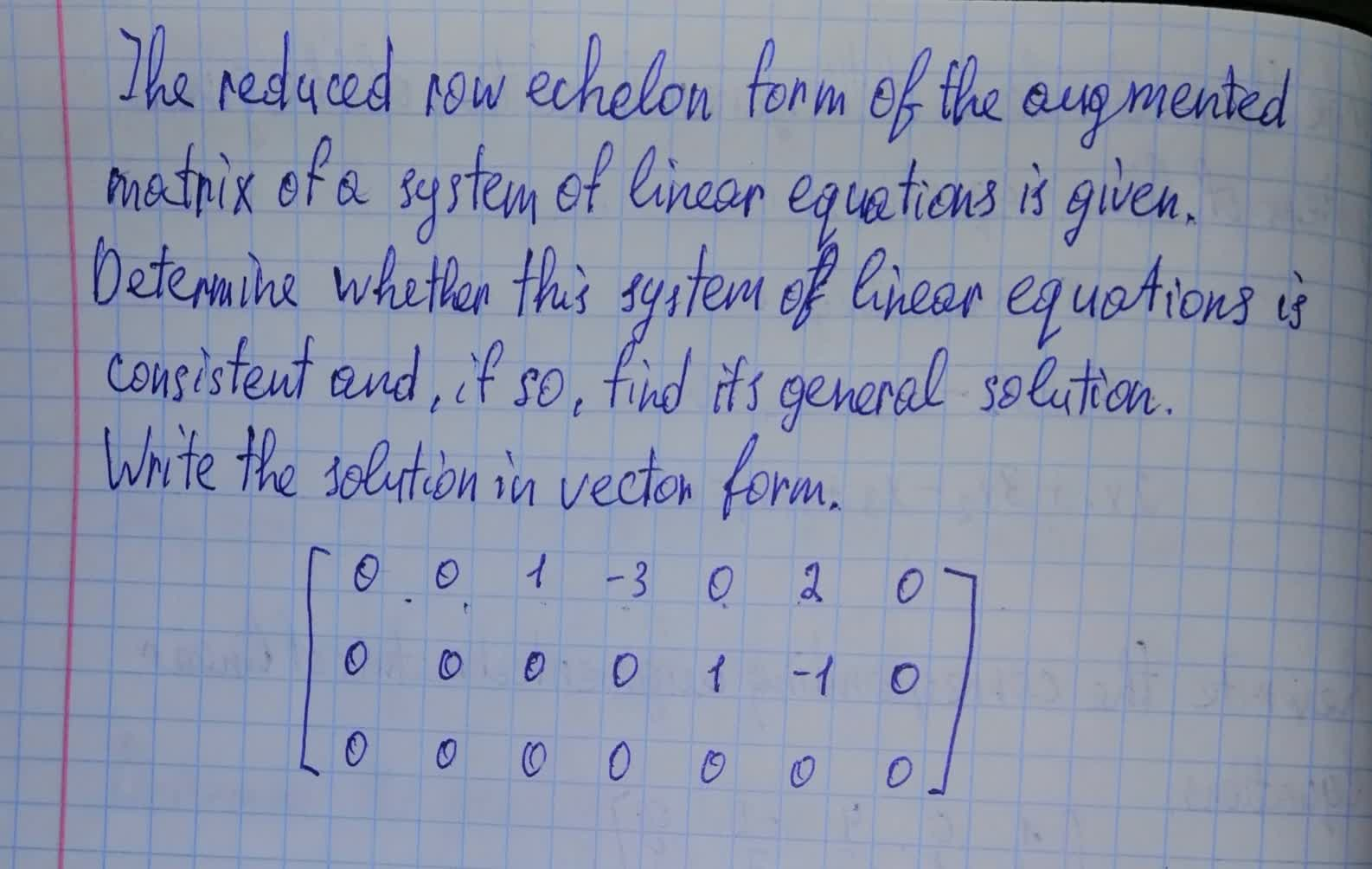Forms of linear equations
ANSWERED### Write the vector form of the general solution of the given system of linear equations. $$\displaystyle{x}_{{1}}+{4}{x}_{{3}}-{2}{x}_{{4}}={0}$$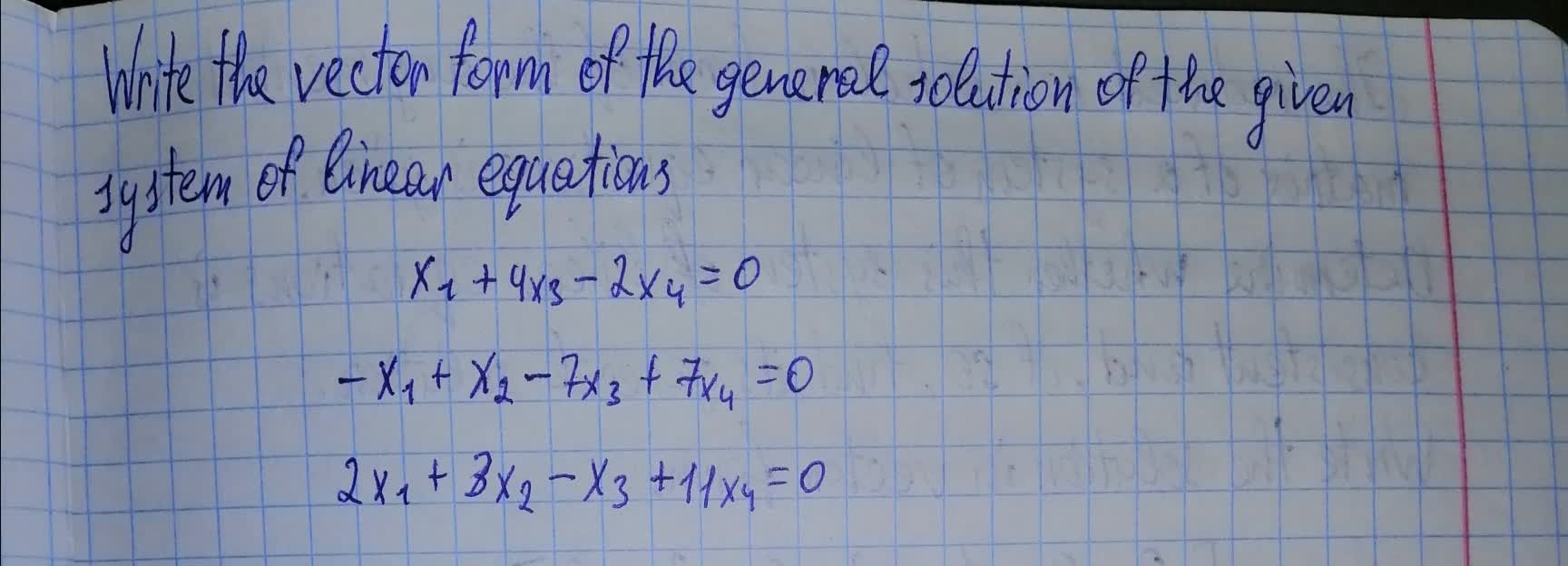Forms of linear equations
ANSWERED### The coefficient matrix for a system of linear differential equations of the form $$\displaystyle{y}^{{1}}={A}_{{y}}$$ has the given eigenvalues and eigenspace bases. Find the general solution for the system.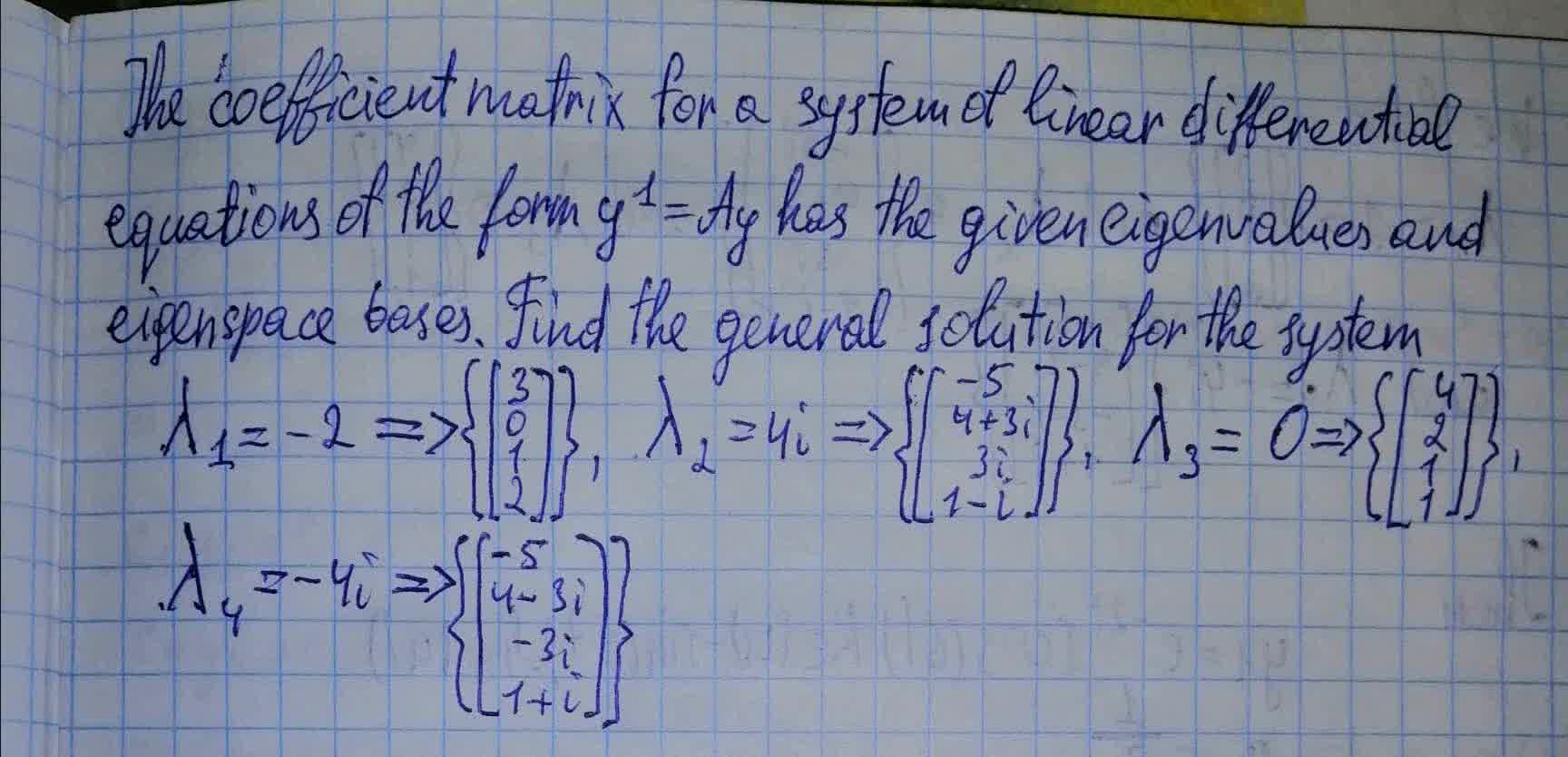Alternate coordinate systems
ANSWERED### This question has to do with binary star systems, where 'i' is the angle of inclination of the system. Calculate the mean expectation value of the factor $$\displaystyle{{\sin}^{{{3}}}}$$ i, i.e., the mean value it would have among an ensemble of binaries with random inclinations. Find the masses of the two stars, if $$\displaystyle{{\sin}^{{{3}}}}$$ i has its mean value. Hint: In spherical coordinates, $$\displaystyle{\left(\theta,\phi\right)}$$, integrate over the solid angle of a sphere where the observer is in the direction of the z-axis, with each solid angle element weighted by $$\sin^3 \theta$$. $$\displaystyle{v}_{{{1}}}={100}{k}\frac{{m}}{{s}}$$ $$\displaystyle{v}_{{{2}}}={200}{k}\frac{{m}}{{s}}$$ Orbital period $$\displaystyle={2}$$ days $$\displaystyle{M}_{{{1}}}={5.74}{e}^{33}{g}$$ $$\displaystyle{M}_{{{2}}}={2.87}{e}^{33}{g}$$

Forms of linear equations
ANSWERED### Write the vector form of the general solution of the given system of linear equations. $$\displaystyle{x}_{{1}}-{x}_{{2}}-{2}{x}_{{4}}-{x}_{{5}}+{4}{x}_{{6}}={0}$$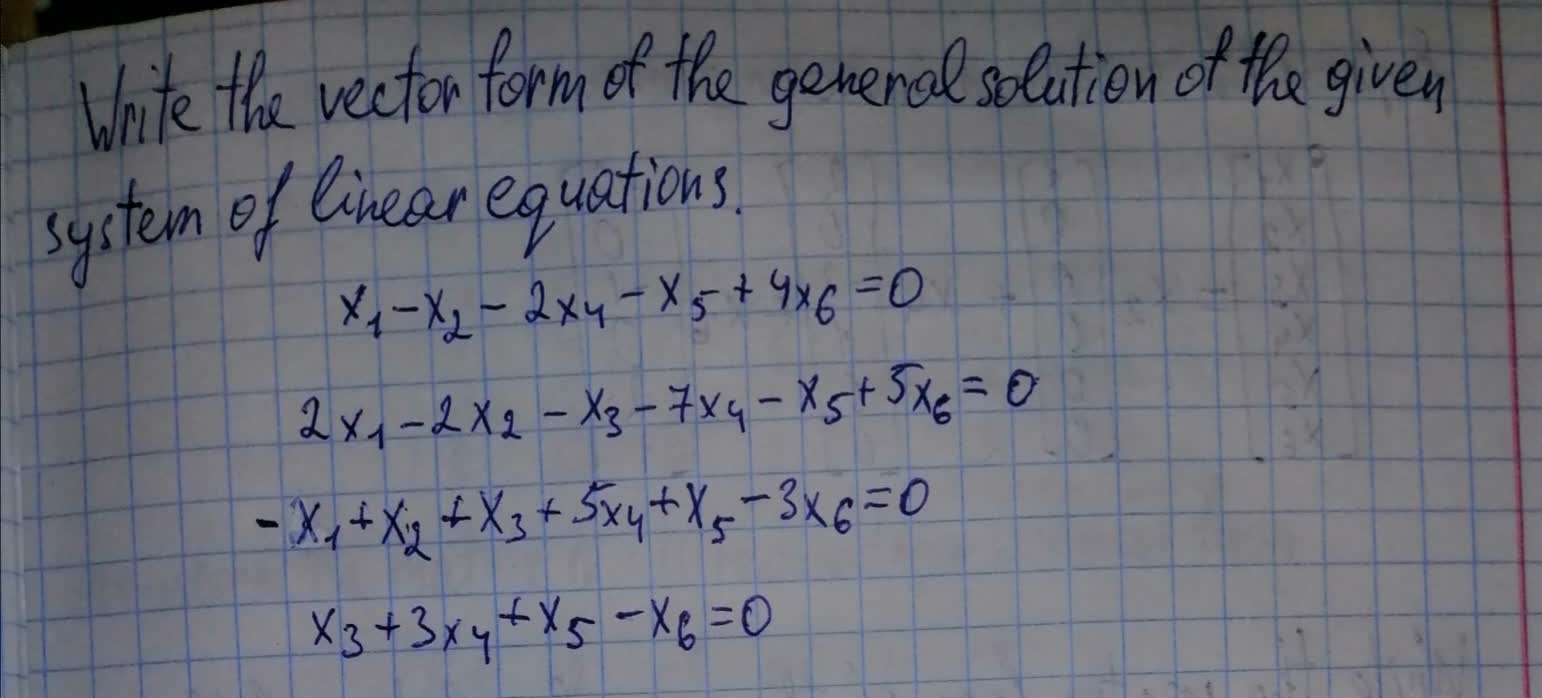Forms of linear equations
ANSWERED### The given matrix is the augmented matrix for a system of linear equations $$\Bigg[ \begin{array}{} 1&0&-1&0&-1&-2&0\\ 0&1&2&0&1&2&0 \\ 0&0&0&1&1&1&0\\ \end{array} \Bigg]$$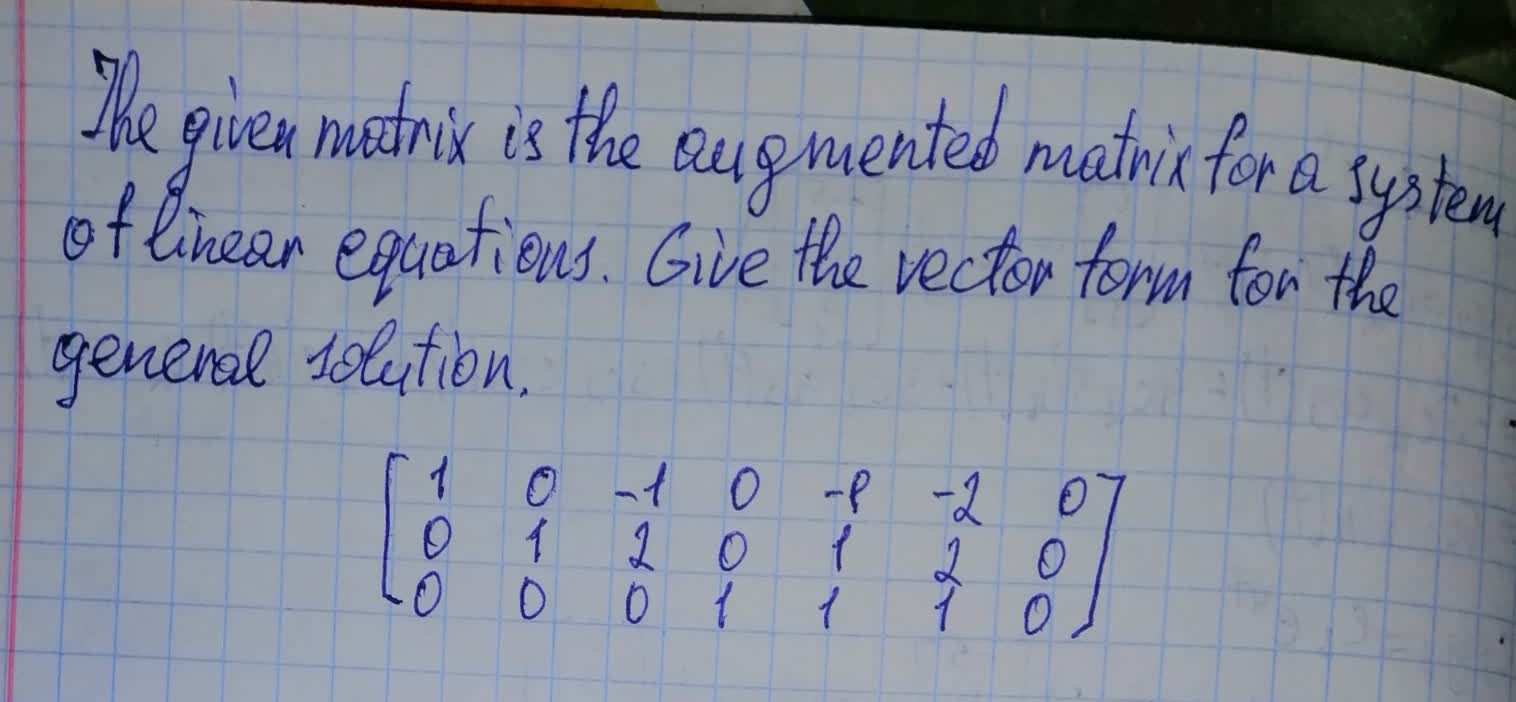Matrix transformations
ANSWERED